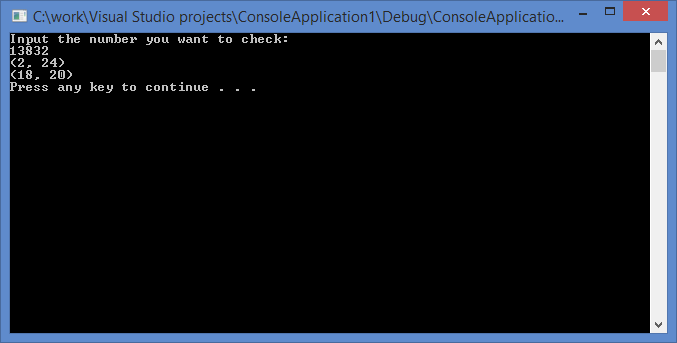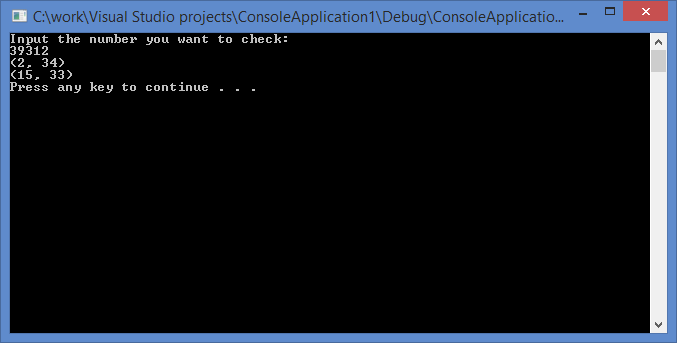# Sum of Two Cubes Example: Finding Cube Pairs

## Assignment:

You have a number x – find a couple of pairs that can represent x as a sum of two cubes.

Example:$x = a^3 + b^3 = c^3 + d^3$$32832 = (4^3 + 32^3)$ or$(18^3 + 30^3)$$39312 = (2^3 + 34^3)$ or$(15^3 + 33^3)$$40033 = (9^3 + 34^3)$ or$(16^3 + 33^3)$

This would be the solutions to the Diophantine equation,$a^3 + b^3 = c^3 + d^3$

Any number x that satisfies the demand will have two pairs$(a, b)$ and$(c, d)$ such that$a, b, c$ and$d$ are all less than$x1/3$. We should create an additional array of size$x1/3$. Every index$i$ in the array will contain the value that equals to the cube of$array[i] = i3$. Now the problem reduces to the search of the pair of elements in the sorted array whose sum is equal to given$x$.

C++ implementation:

```#include <iostream>
#include <cmath>
using namespace std;

void searchPairs(int n)
{
int root = pow(n, 1.0 / 3.0);

int *cube = new int[root + 1];

for (int i = 1; i <= root; i++)
cube[i] = i*i*i;

int a = 1;
int b = root;

while (a < b)
{
if (cube[a] + cube[b] < n) a++; else if (cube[a] + cube[b] > n)
b--;
else {
cout << "(" << a << ", " << b
<< ")" << endl;
a++; b--;
}
}
}

int main()
{
int n = 0;
cout << "Input the number you want to check: \n"; cin >> n;

searchPairs(n);

system("pause");
return 0;
}
```

Screenshots of the working program:The presented piece of writing is a sample assignment for the solution of the problem of the sum of two cubes. You can also be interested in our quicksort example. We wish to remind you that you are not allowed to use any of the information contained within or parts of the assignments themselves which are posted on our blog without proper references to the source, since it will be considered as a violation of the author’s rights, and thus plagiarism. More than that, it’s a very ineffective way of dealing with your academic problems, because you can place an order and simply wait until our professionals work on your assignment.

To place an order, first fill in the short order form at the top of the page, where you need to provide your e-mail and the discipline of your assignment. After that, you will be taken to the page with a more detailed form. Specify your requirements, upload any additional files and wait for our experts to start bidding for your order. You can choose an expert you like after reviewing his or her personal profile. A typical expert’s profile contains information about the previous orders, average rating and feedback from customers. Choose the one who is the best fit for solving your sum of two cubes problem and wait for the paper to be done for you. Learning will become easy with our experts!